# 一种对缆索无损探伤微弱信号的处理方法A Weak Signal Process Method of Cable Nondestructive Inspection

DOI: 10.12677/HJCE.2019.83091, PDF, HTML, XML, 下载: 274  浏览: 399

Abstract: A method is designed to deal with the problem that the signal-to-noise ratio of the weak signal is small when processing the weak signal of nondestructive inspection of cables, which can’t clearly reflect the tiny defects inside the cables. This method collects the weak signal several times under the accurate timing control and accumulates the collected signal sequence strictly according to the time line. Because the distribution of signal noise in time domain belongs to normal distribution, i.e. uniform and symmetrical distribution on both sides of the real value will cancel each other after accumulating, while the real signal does not belong to normal distribution. After multiple ac-cumulations, the signal value will be amplified. By this method, the real signal can be amplified ef-fectively besides the noise, so that the weak signal generated by the weak internal defects can be clearly distinguished, and the accuracy of nondestructive inspection is greatly improved. This method is simple, easy to operate and has a wide range of applications, and has high popularization value.

1. 引言

2. 缆索无损探伤系统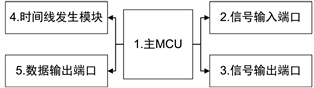Figure 1. Hardware diagram of cable NDT system

3. 信号处理

$p\left(x\right)=\frac{1}{\sqrt{2\pi }\sigma }\mathrm{exp}\left\{-\frac{{\left(x-\mu \right)}^{2}}{2{\sigma }^{2}}\right\}$ (1)

$F\left(x\right)=\frac{1}{\sqrt{2\text{π}}\sigma }{\int }_{-\infty }^{x}\mathrm{exp}\left\{-\frac{{\left(x-\mu \right)}^{2}}{2{\sigma }^{2}}\right\}\text{d}x$ (2)

$E\left(x\right)=\frac{1}{\sqrt{2\text{π}}\sigma }{\int }_{-\infty }^{+\infty }x\mathrm{exp}\left\{-\frac{{\left(x-\mu \right)}^{2}}{2{\sigma }^{2}}\right\}\text{d}x$ (3)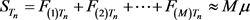(4)

${S}_{\left[{T}_{1}\text{}{T}_{2}\text{}\cdots {T}_{n}\right]}=\left[\begin{array}{ccc}{F}_{\left(1\right){T}_{1}}& \cdots & {F}_{\left(1\right){T}_{n}}\\ ⋮& \ddots & ⋮\\ {F}_{\left(M\right){T}_{1}}& \cdots & {F}_{\left(M\right){T}_{n}}\end{array}\right]$ (5)

${S}_{\left[{T}_{1}\text{}{T}_{2}\text{}\cdots {T}_{n}\right]}=\left[M{\mu }_{1}M{\mu }_{2}\cdots M{\mu }_{n}\right]$ (6)

1) 由图1中的4产生时间线，作为整个流程的时序基准。

2) 在T1时刻，由图1中的2产生发射脉冲信号，并等待50 ms。

3) 等时间间隔，从图1中的3采集回波信号序列，保存为 $\left({F}_{1},{F}_{2},\cdots {F}_{n}\right)$

4) 重复2~3步骤M次，得到 ${\left({F}_{1},{F}_{2},\cdots {F}_{n}\right)}_{1}$ ~ ${\left({F}_{1},{F}_{2},\cdots {F}_{n}\right)}_{M}$ ，M组信号序列。

5) 将M组信号序列按照进行矩阵相加运算，得到结果序列。对结果序列进行归一化，得到最终结果序列数据。

6) 将结果序列通过图1中的5进行数据输出，显示成回波曲线，方便测试人员判断结果。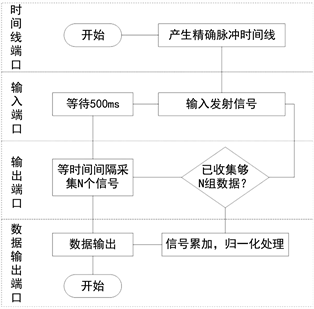Figure 2. Signal process flow chart

4. 实验结果分析Table 1. Signal processing results of coefficients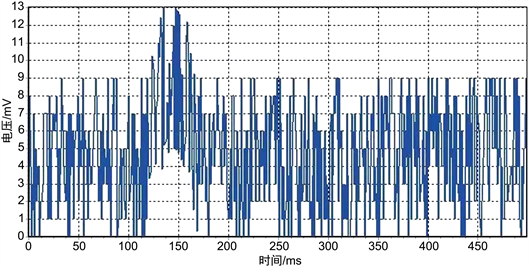Figure 3. Original signal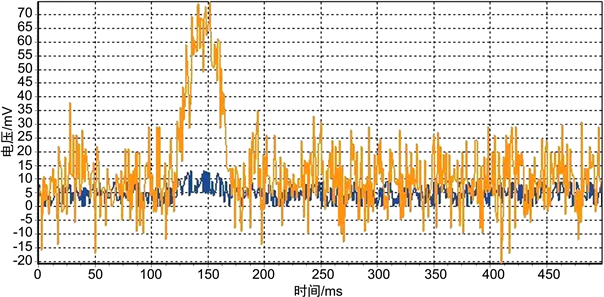Figure 4. Processed signal with coefficient 10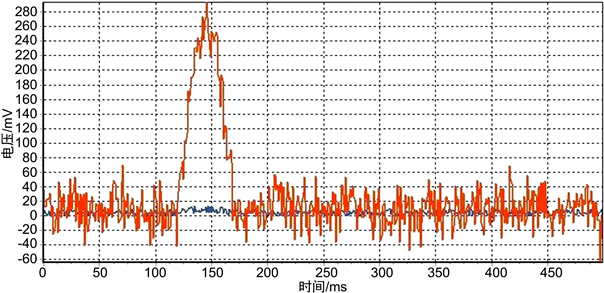Figure 5. Processed signal with coefficient 50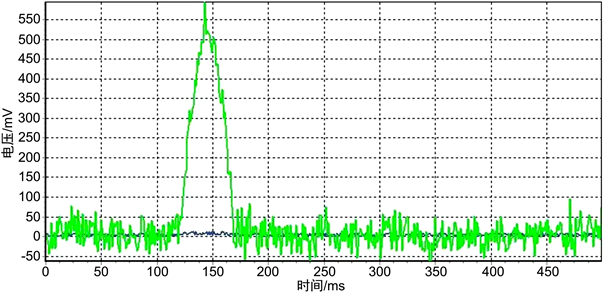Figure 6. Processed signal with coefficient 100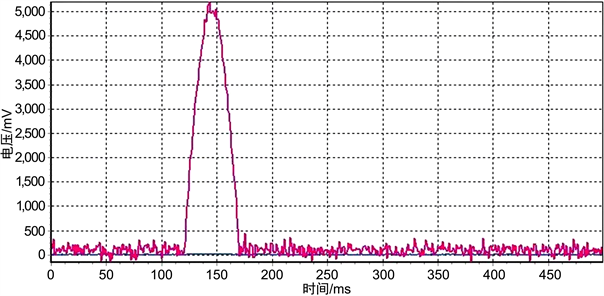Figure 7. Processed signal with coefficient 1000

5. 结束语

  邱宇, 朱承建. 钢丝绳无损探伤仪研究[J]. 电子世界, 2013(13): 128-130.  宋静. 手持式超声波探伤仪应用软件设计[D]: [硕士学位论文]. 南京: 南京航空航天大学, 2009.  董谦, 谢剑英. 超声波无损检测系统的噪声干扰分析与抑制[J]. 测控技术, 2001, 20(11): 50-52.  赵文礼, 夏炜, 刘鹏, 等. 基于混沌理论的微弱信号放大原理与方法研究[J]. 物理学报, 2010, 59(5): 2962-2970.  朱勇辉, 朱其猛. 超声波应力测试降噪技术[J]. 电焊机, 2018, 48(2): 105-107.  彭瑾, 王黎, 高晓蓉, 等. 基于相位分析的超声波噪声信号抑制算法[J]. 铁道技术监督, 2009, 37(1): 34-37.  徐灵活, 殷明, 章建程, 等. 正态分布法在噪声听觉损伤易感性评价中的应用探讨[J]. 海军医学杂志, 2007, 28(1): 5-7.  王培容, 杨艺. 统计平均拟合正态分布曲线的迭代算法及其应用[J]. 重庆工商大学学报(自然科学版), 2001, 18(1): 37-40.  金秋, 刘少岗. 线性不对称损失时非正态分布过程均值优化[J]. 运筹与管理, 2014(6): 23-28.  辛士波. 基于双侧等截尾正态分布的均值-极差控制图[J]. 火力与指挥控制, 2012, 37(8): 180-183.